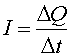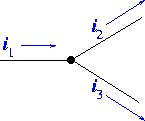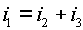Currents, Amperes, ... Current in a circuit is a measure of the flow of charge.where DQ is the amount of charge that flows by in a time Dt. The unit of current is Amperes or amps. One amp is equivalent to one Coulomb per second. Charge is a conserved quantity which can not be created or destroyed, therefore the current which flows into a junction must equal the current that flows out. For instance, if three wires are attached to a node as shown below,